A high leverage data point has extreme predictor $$X$$ values. An outlier is a data point whose response value $$y$$ does not follow the general trend of the rest of the data. A data point is influential if it unduly influences predicted responses, the estimated slope coefficients, or hypothesis test results. High leverage data points and outliers may or may not be influential - you need to investigate!

If you have influential data points, you can 1) do nothing because there is theoretical reasons to include them, 2) drop them, or 3) include them with reduced weight using robust regression.

# High Leverage Points

The “hat matrix” $$H$$ identifies any leverage points. Recall that in the linear regression model $$\hat{y} = X \hat{\beta}$$ the slope coefficients are estimated by $$\hat{\beta} = (X'X)^{-1}X'y$$. Substituting, $$\hat{y} = X(X'X)^{-1}X'y$$, or $$\hat{y} = Hy$$, where

$H = X(X'X)^{-1}X'.$

$$H$$ is called the hat matrix because $$H$$ puts the hat on $$y$$. $$H$$ is an $$n \times n$$ matrix. The diagonal elements $$H_{ii}$$ are a measure of the distances between each observation $$i$$’s predictor variables $$X_i$$ and the average of the entire data set predictor variables $$\bar{X}$$. $$H_{ii}$$ are the leverage that the observed responses $$y_i$$ exert on the predicted responses $$\hat{y}_i$$. Each $$H_{ii}$$ is in the unit interval [0, 1] and the values sum to the number of regression parameters (including the intercept) $$\sum{H_{ii}} = p$$. A common rule is to research any observation whose leverage value is more than 3 times larger than the mean leverage value, which since the sum of the leverage values is $$p$$, equals

$H_{ii} > 3 \frac{p}{n}.$

In practice, you will identify and deal with influential data points, not the leverage points that give rise to them. However, since leverage is an important component of influential data points, you should know how to work with it.

#### Example

A study measured cement hardening based on its chemical composition. Data set cement (cement.txt) is a summary of n = 13 formulations:

• y = heat evolved, in calories/gram
• x1 = % of tricalcium aluminate
• x2 = % of tricalcium silicate
• x3 = % of tetracalcium alumino ferrite
• x4 = % of dicalcium silicate
library(readr)
skip = 1,
fwf_widths(c(5, 7, 7, 7, 7)))
colnames(cement) <- c("y", "x1", "x2", "x3", "x4")
head(cement)
## # A tibble: 6 x 5
##       y    x1    x2    x3    x4
##   <dbl> <dbl> <dbl> <dbl> <dbl>
## 1  78.5     7    26     6    60
## 2  74.3     1    29    15    52
## 3 104.     11    56     8    20
## 4  87.6    11    31     8    47
## 5  95.9     7    52     6    33
## 6 109.     11    55     9    22

Suppose you model y ~ x1.

cement_lm1 <- lm(y ~ x1, data = cement)

From the scatterplot it looks like observation 10 may be a high leverage point.

library(ggplot2)
ggplot(data = cement, aes(y = y, x = x1)) +
geom_point() +
geom_smooth(method = "lm", se = FALSE) +
labs(title = "y ~ x1",
subtitle = "Obs 10 a high leverage point?") +
geom_text(label=rownames(cement),
nudge_x = 0.5,
nudge_y = 0.25,
check_overlap = T)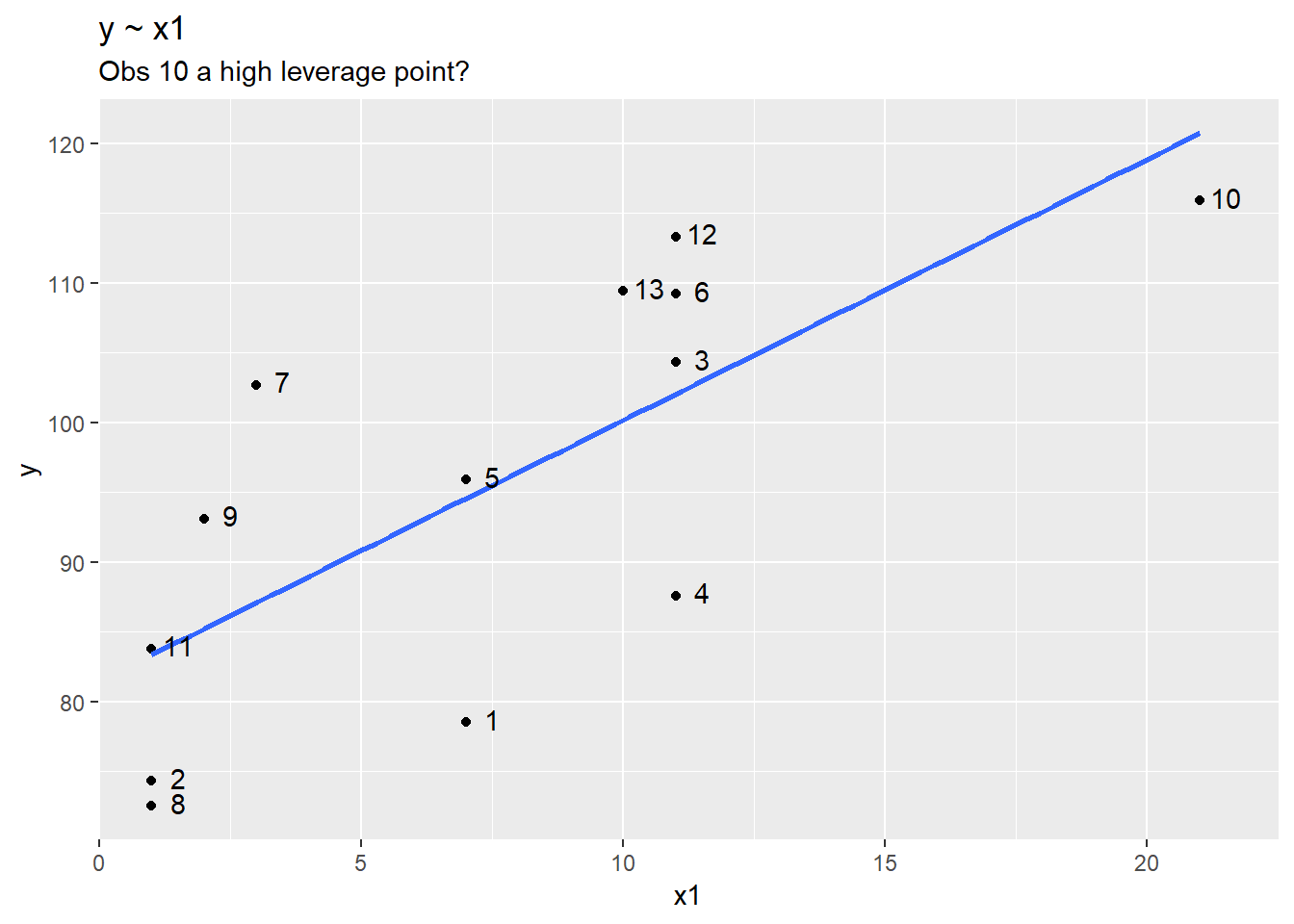The hat matrix is $$H = X(X'X)^{-1}X'$$. The diagonal elements are the leverages. The further each $$X_i$$ is from $$\bar{X}$$, the larger the leverage. Observation 10 has the largest leverage.

X <- matrix(c(rep(1, times = nrow(cement)),
cement$x1), ncol = 2) # t(x) is transpose of x: x' # solve(x) is inverse of x: x^{-1} H <- X %*% solve(t(X) %*% X) %*% t(X) data.frame(x1 = cement$x1,
H = diag(H))
##    x1          H
## 1   7 0.07743609
## 2   1 0.17747314
## 3  11 0.10707670
## 4  11 0.10707670
## 5   7 0.07743609
## 6  11 0.10707670
## 7   3 0.12486106
## 8   1 0.17747314
## 9   2 0.14875880
## 10 21 0.51834013
## 11  1 0.17747314
## 12 11 0.10707670
## 13 10 0.09244165

The sum of the matrix diagonal (trace) equals the number of predictor variables in the model.

sum(diag(H))
##  2

You did not need manually calculate the hat matrix for this exercise becaues R function hatvalues(model) returns the diagonal elements of the hat matrix. Which observation(s) are high leverage points? Observation 10 is more than three times the mean leverage.

outliers <- hatvalues(cement_lm1) > 3 * mean(hatvalues(cement_lm1))
cement[outliers, ]
## # A tibble: 1 x 5
##       y    x1    x2    x3    x4
##   <dbl> <dbl> <dbl> <dbl> <dbl>
## 1  116.    21    47     4    26

For multiple linear regression models like y ~ x2 + x2 + x3 + x4, there is no straight-forward visual diagnostic like the simple linear regression scatterplot. However, the method is otherwise unchanged. In this case, there are no outliers.

cement_lm2 <- lm(y ~ x1 + x2 + x3 + x4,
data = cement)

outliers <- hatvalues(cement_lm2) > 3 * mean(hatvalues(cement_lm2))
cement[outliers, ]
## # A tibble: 0 x 5
## # ... with 5 variables: y <dbl>, x1 <dbl>, x2 <dbl>, x3 <dbl>, x4 <dbl>

# Outliers

An outlier is a point whose observation is far from the fitted regression line. That is, an outlier is a point with a large residual. “Large” is relative to the variable units, so standardize the residuals by dividing by an estimate of their standard deviation.1 Residuals standardized in this way are sometimes called internally standardized residuals or internally studentized residuals.

$r_i = \frac{\epsilon_i}{\sqrt{MSE(1 - H_{ii})}}$

mse <- sum(cement_lm2$residuals^2) / df.residual(cement_lm2) cement_lm2$residuals / sqrt(mse*(1 - hatvalues(cement_lm2)))
##            1            2            3            4            5
##  0.002902141  0.756624558 -1.050274056 -0.841081415  0.127905849
##            6            7            8            9           10
##  1.714815620 -0.744450296 -1.687801801  0.670799981  0.210293420
##           11           12           13
##  1.073910078  0.463352296 -1.124105189

These residuals are calculated by the rstandard() function.

rstandard(cement_lm2)
##            1            2            3            4            5
##  0.002902141  0.756624558 -1.050274056 -0.841081415  0.127905849
##            6            7            8            9           10
##  1.714815620 -0.744450296 -1.687801801  0.670799981  0.210293420
##           11           12           13
##  1.073910078  0.463352296 -1.124105189

An observation with a standardized residual that is larger than 3 is generally considered an outlier. One problem with this definition is that potential outlying observations influence the slope of regression line, muting their measured residual. A second definition of an outlier using the externally studentized residual remedies this problem. Externally standardized (studentized) residuals are the residuals divided by the MSE measured with observation $$i$$ removed, $$MSE_{(i)}$$.

$r_i = \frac{\epsilon_i}{\sqrt{MSE_{(i)}(1 - H_{ii})}}$

Calculating $$MSE_i$$ is a process of leave-one-out regression diagnostics. In the code below, mdl is a list of $$i = 1:n$$ models with observation i removed. mse is the resulting mean squared error, $$\sum{\epsilon^2} / df_\epsilon$$.

library(purrr)
i <- 1:13
mdl <- map(i, function(i) lm(y ~ x1 + x2 + x3 + x4,
data = cement[-i, ]))
mse <- as.numeric(map(i, function(i) sum(mdl[[i]]$residuals^2) / mdl[[i]]$df.residual))
as.numeric(map(i, function(i)  (cement_lm2$residuals / sqrt(mse[[i]] * (1 - hatvalues(cement_lm2))))[i])) ##  0.002714706 0.734526654 -1.058093203 -0.824036397 0.119767490 ##  2.017049821 -0.721820523 -1.967482994 0.645903738 0.197257449 ##  1.085864774 0.439362041 -1.145888712 These residuals are calculated by the rstudent(model) function. rstudent(cement_lm2) ## 1 2 3 4 5 ## 0.002714706 0.734526654 -1.058093203 -0.824036397 0.119767490 ## 6 7 8 9 10 ## 2.017049821 -0.721820523 -1.967482994 0.645903738 0.197257449 ## 11 12 13 ## 1.085864774 0.439362041 -1.145888712 # Influential Points There are two common measures for identifying influential data points: difference in fits (DFFITS), and Cook’s distance. Both methods use the leave-one-out process from above. ## DFFITS The difference in fits for obseravation $$i$$ is defined $DFFITS_i = \frac{\hat{y}_i - \hat{y}_{(i)}}{\sqrt{MSE_{(i)} H_{ii}}}.$ $$DFFITS$$ is similar to the externally standardized residual definition, except that the numerator is the difference in fitted values with observation $$i$$ and without observation $$i$$. An observation is considered influential if $2 \sqrt{\frac{p + 1}{n - p - 1}}.$ #### Example Data set influence3 (influence3.txt]) has one influential observation. library(dplyr) ## ## Attaching package: 'dplyr' ## The following objects are masked from 'package:stats': ## ## filter, lag ## The following objects are masked from 'package:base': ## ## intersect, setdiff, setequal, union influence3 <- read_tsv(file = "./Data/influence3.txt") ## Parsed with column specification: ## cols( ## Row = col_double(), ## x = col_double(), ## y = col_double() ## ) print(influence3) ## # A tibble: 21 x 3 ## Row x y ## <dbl> <dbl> <dbl> ## 1 1 0.1 -0.0716 ## 2 2 0.454 4.17 ## 3 3 1.10 6.57 ## 4 4 1.28 13.8 ## 5 5 2.21 11.5 ## 6 6 2.50 13.0 ## 7 7 3.04 20.2 ## 8 8 3.24 17.6 ## 9 9 4.45 26.0 ## 10 10 4.17 22.8 ## # ... with 11 more rows From the scatterplot, you can see observation 21 is a high leverage data point. ggplot(data = influence3, aes(y = y, x = x)) + geom_point() + geom_smooth(method = "lm", se = FALSE) + labs(title = "y ~ x", subtitle = "Obs 21 an influential data point?") + geom_text(label=rownames(influence3), nudge_x = 0.5, nudge_y = 0.25, check_overlap = T)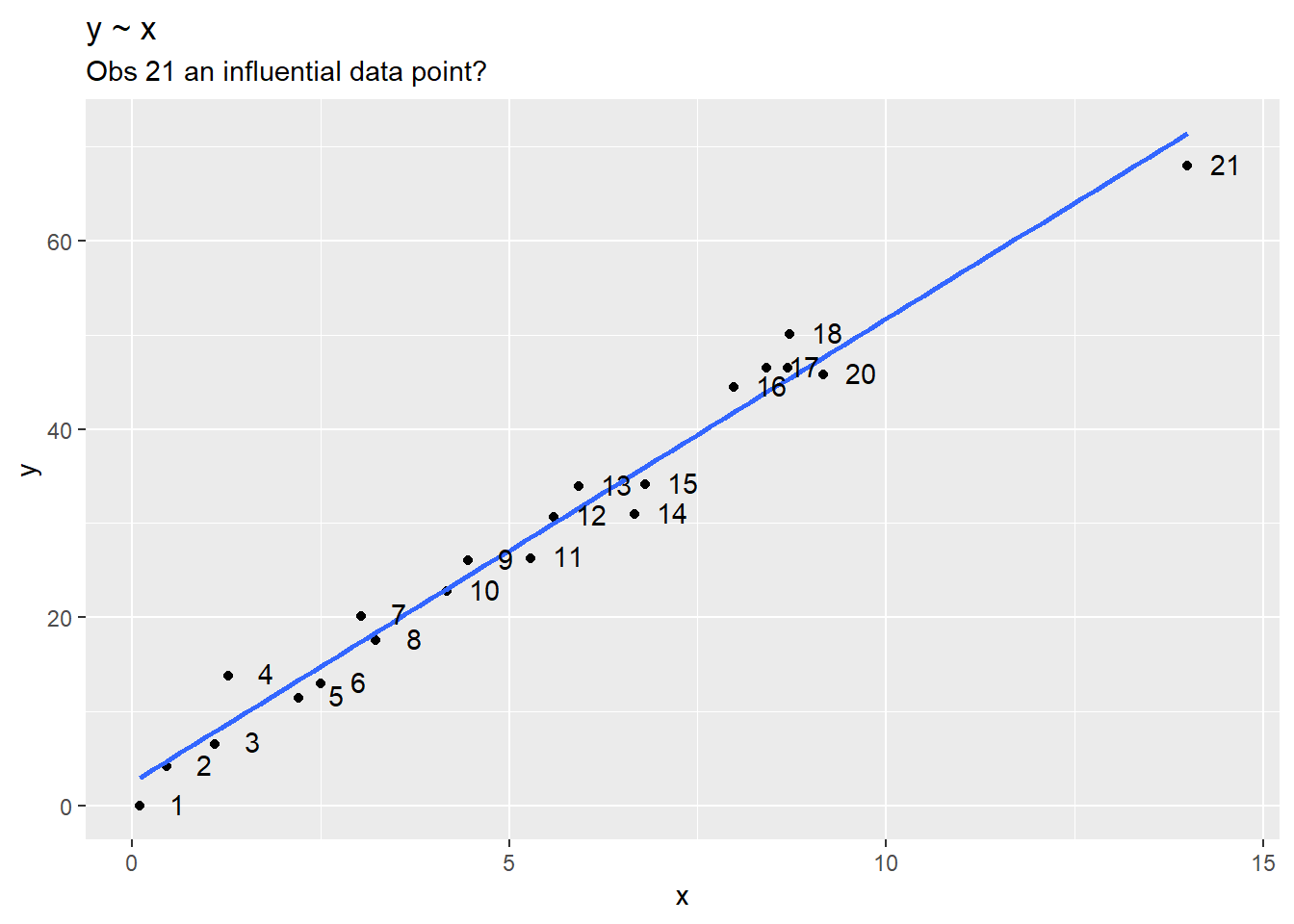That intuition is confirmed by the diagnostic test: influence3_lm <- lm(y ~ x, data = influence3) leverages <- hatvalues(influence3_lm) > 3 * mean(hatvalues(influence3_lm)) influence3[leverages, ] ## # A tibble: 1 x 3 ## Row x y ## <dbl> <dbl> <dbl> ## 1 21 14 68 Is it also an outlier? No! outliers <- rstudent(influence3_lm) > 3 influence3[outliers, ] ## # A tibble: 0 x 3 ## # ... with 3 variables: Row <dbl>, x <dbl>, y <dbl> However, it is potentially influential according to DFFITS. Use the dffits(model) function to calculate the $$DFFITS_i$$ values. In this case look for $$DFFITS_i$$ values (in absolute value) greater than $2 \sqrt{\frac{p + 1}{n - p - 1}} = 2 \sqrt{\frac{2 + 1}{21 - 2 - 1}} = 0.82.$ Observation 21 is a potential influential data point. df <- influence3_lm$df.residual
p <- length(influence3_lm$coefficients) n <- nrow(influence3_lm$model)
dffits_crit = 2 * sqrt((p + 1) / (n - p - 1))
influence3_dffits <- dffits(influence3_lm)
df <- data.frame(obs = names(influence3_dffits),
dffits = influence3_dffits)
ggplot(df, aes(y = dffits, x = obs)) +
geom_point() +
geom_hline(yintercept = c(dffits_crit, -dffits_crit), linetype="dashed") +
labs(title = "DFFITS",
subtitle = "Influential Observation at observation 21.",
x = "Observation Number",
y = "DFFITS")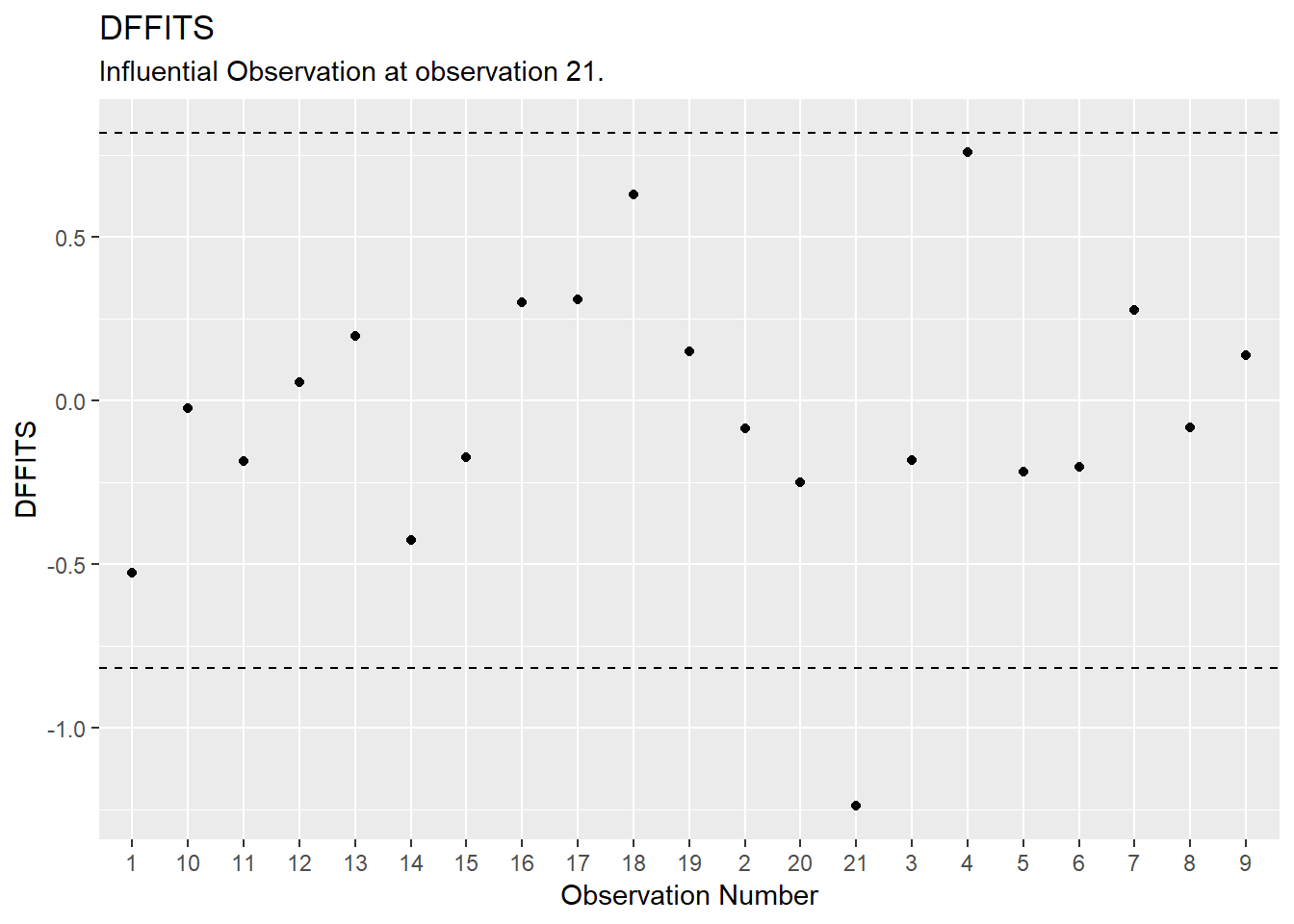influence3[which(abs(influence3_dffits) > dffits_crit),]
## # A tibble: 1 x 3
##     Row     x     y
##   <dbl> <dbl> <dbl>
## 1    21    14    68

This is surprising because it certainly seems to fit within the data set well. Remember, DFFITS just flags potentially influential data points - you should check whether the potential influential data points significantly alter the outcome of the regression analysis. If they do, report the results with and without the influential points.

## Cook’s Distance

Cook’s distance for observation $$i$$ is defined

$D_i = \frac{(y_i - \hat{y}_i)^2}{p \times MSE} \frac{H_{ii}}{(1 - H_{ii})^2}.$

$$D_i$$ directly summarizes how much all of the fitted values change when the ith observation is deleted. ???A data point with $$D_i > 1$$ is probably influential. $$D_i > 0.5$$ is at least worth investigating.

Using Cook’s distance, observation 21 is still potentially influential.

cooks_crit = 0.5
influence3_cooks <- cooks.distance(influence3_lm)
df <- data.frame(obs = names(influence3_cooks),
cooks = influence3_cooks)
ggplot(df, aes(y = cooks, x = obs)) +
geom_point() +
geom_hline(yintercept = cooks_crit, linetype="dashed") +
labs(title = "Cook's Distance",
subtitle = "Influential Observation at observation 21.",
x = "Observation Number",
y = "Cook's")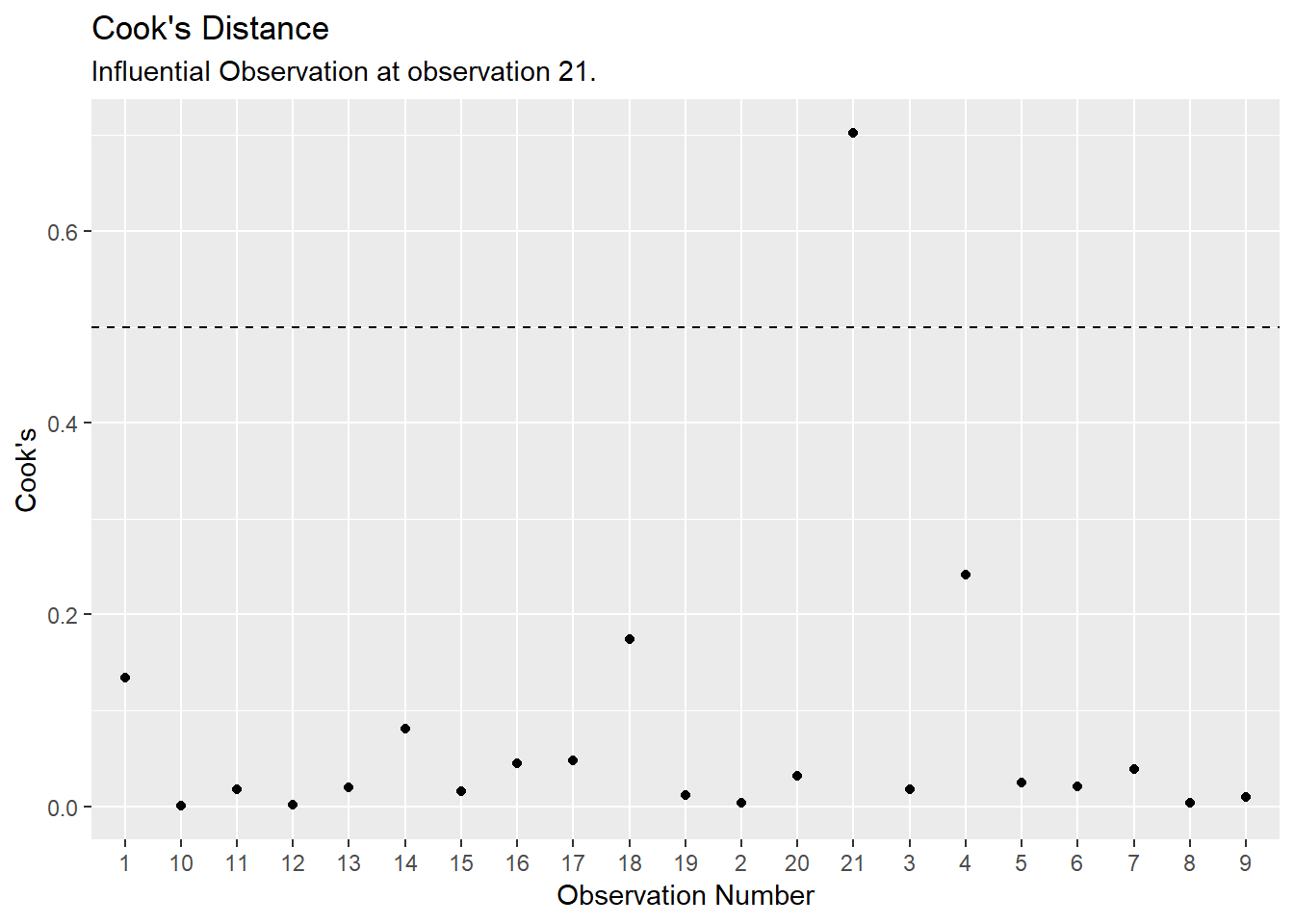influence3[which(abs(influence3_cooks) > cooks_crit),]
## # A tibble: 1 x 3
##     Row     x     y
##   <dbl> <dbl> <dbl>
## 1    21    14    68

# Full Example

Dataset allentest (allentest.txt2) contains Allen Cognitive Level scores and three test scores.

• ACL: Allen Cognitive Level score
• Vocab: vocabulary test score
• Abstract: Abstraction test score
• SDMT: SDMT test score
allentest <- read_tsv(file = "./Data/allentest.txt")
head(allentest)
## # A tibble: 6 x 5
##    Subj   ACL Vocab Abstract  SDMT
##   <dbl> <dbl> <dbl>    <dbl> <dbl>
## 1     1   6      28       36    70
## 2     2   5.4    34       32    49
## 3     3   4.7    19        8    28
## 4     4   4.8    32       28    47
## 5     5   4.9    22        4    29
## 6     6   4.5    24       24    23

The scatterplot matrix suggests which predictors to include in the model. ACL is most strongly correlated with SDMT, but less so with Abstract and Vocab. SDMT is also strongly correlated with both the other predictor variables Abstract and Vocab.

plot(allentest[, -1])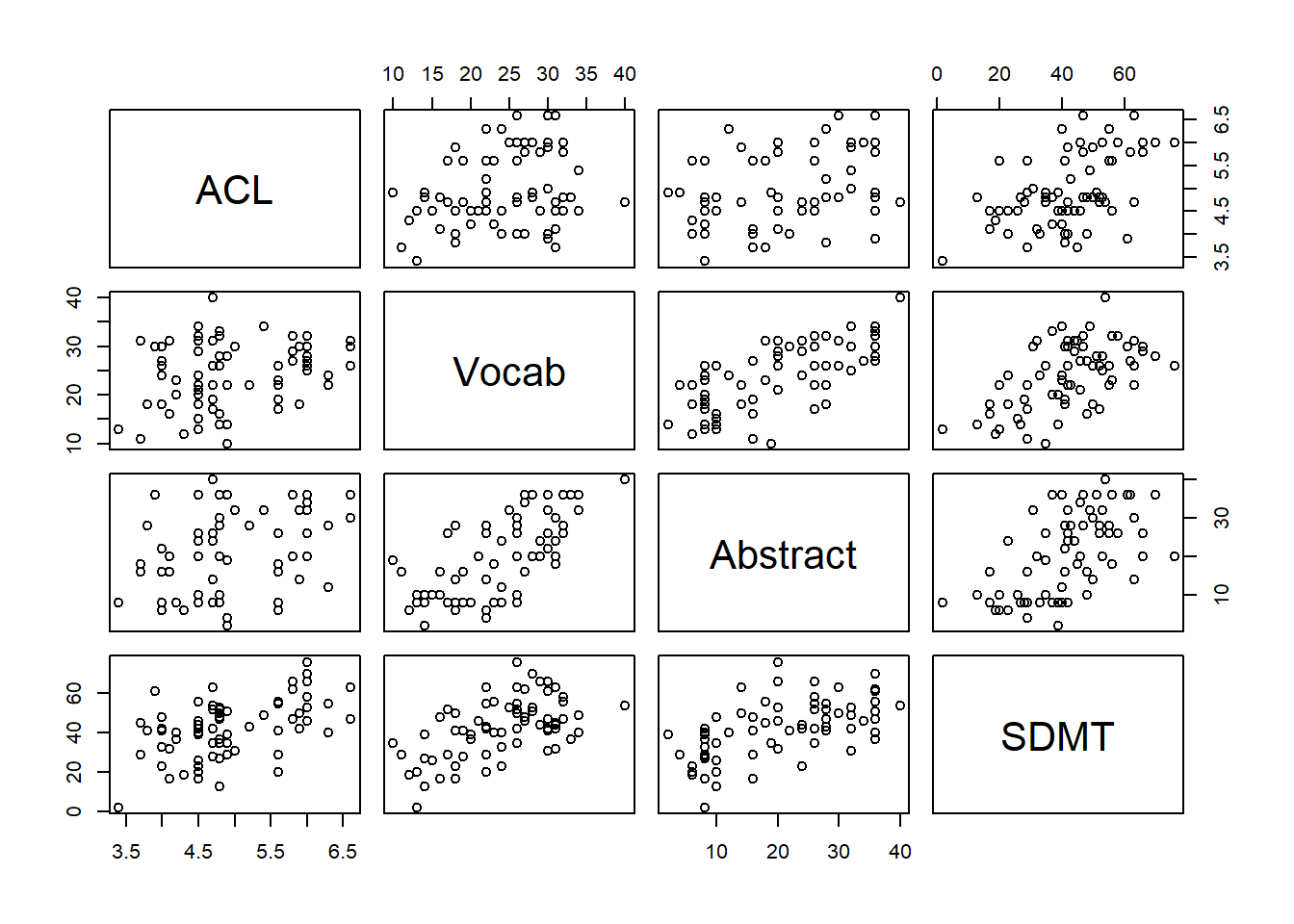cor(allentest[,-1], method = "pearson")
##                ACL     Vocab  Abstract      SDMT
## ACL      1.0000000 0.2500002 0.3537383 0.5208768
## Vocab    0.2500002 1.0000000 0.6978405 0.5560707
## Abstract 0.3537383 0.6978405 1.0000000 0.5769238
## SDMT     0.5208768 0.5560707 0.5769238 1.0000000
library(corrplot)
corrplot(cor(allentest[,-1], method = "pearson"))